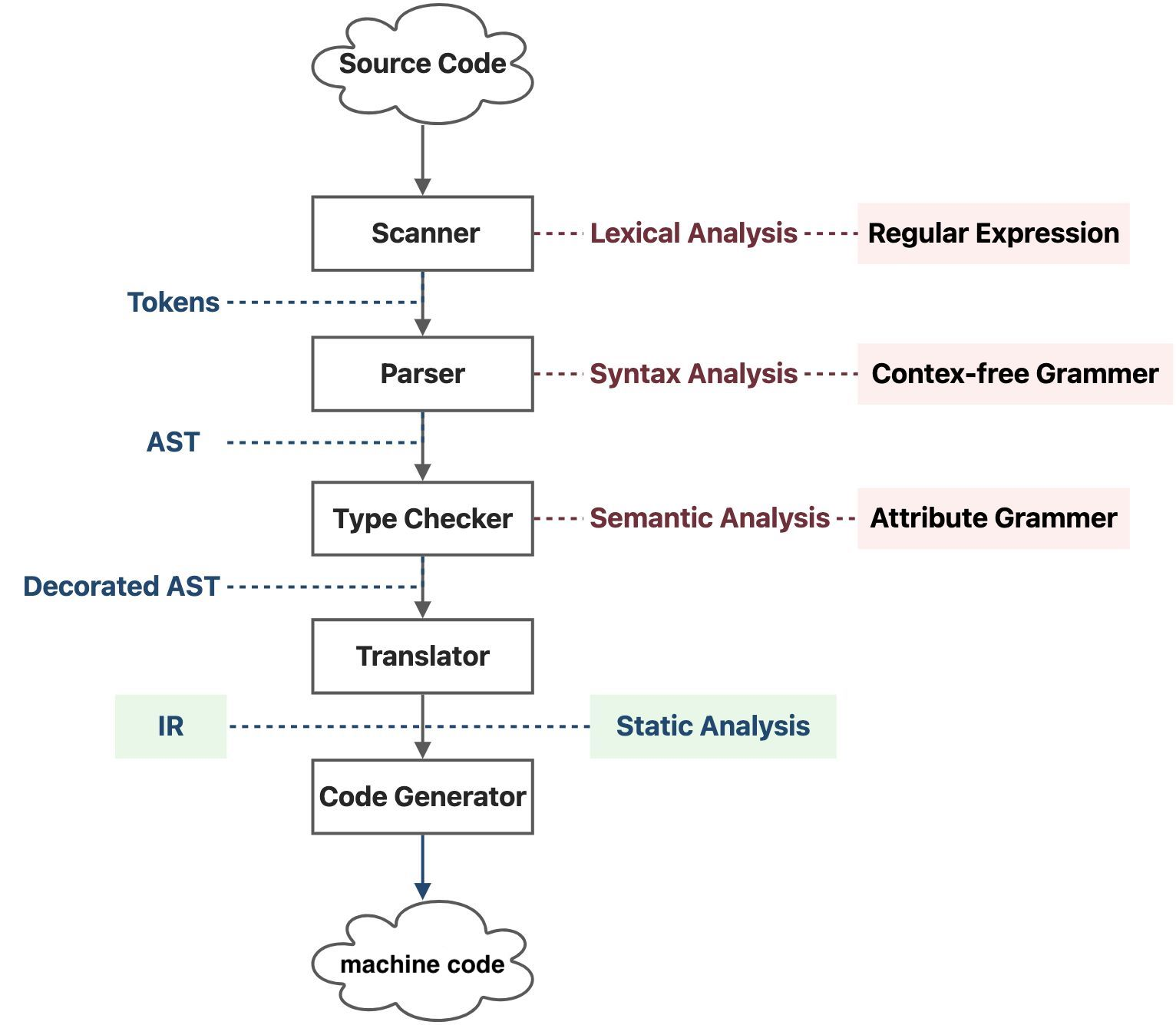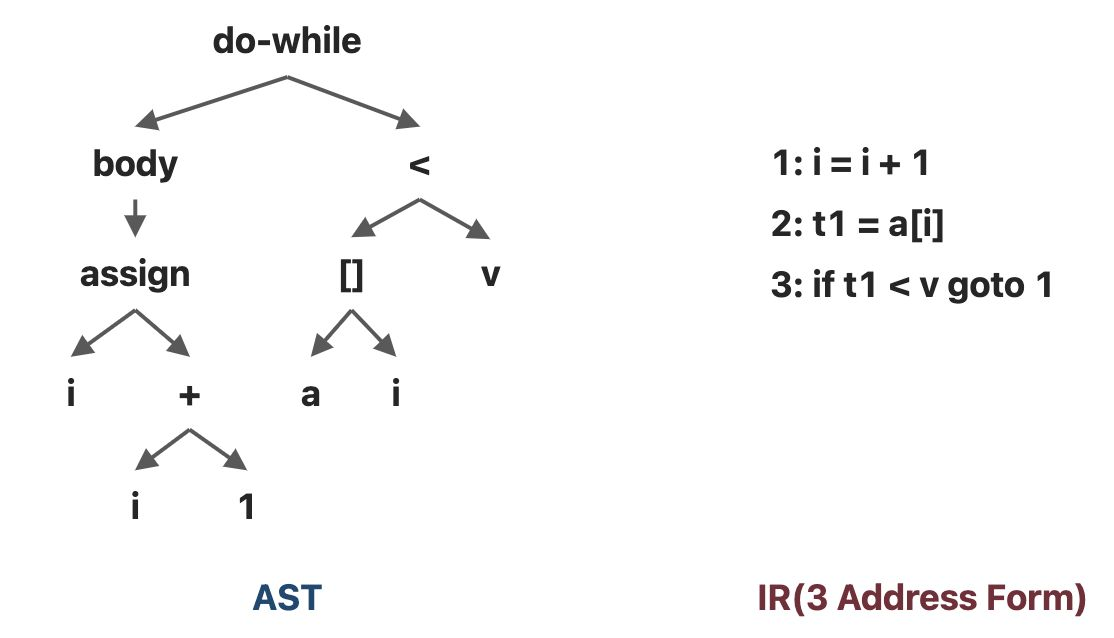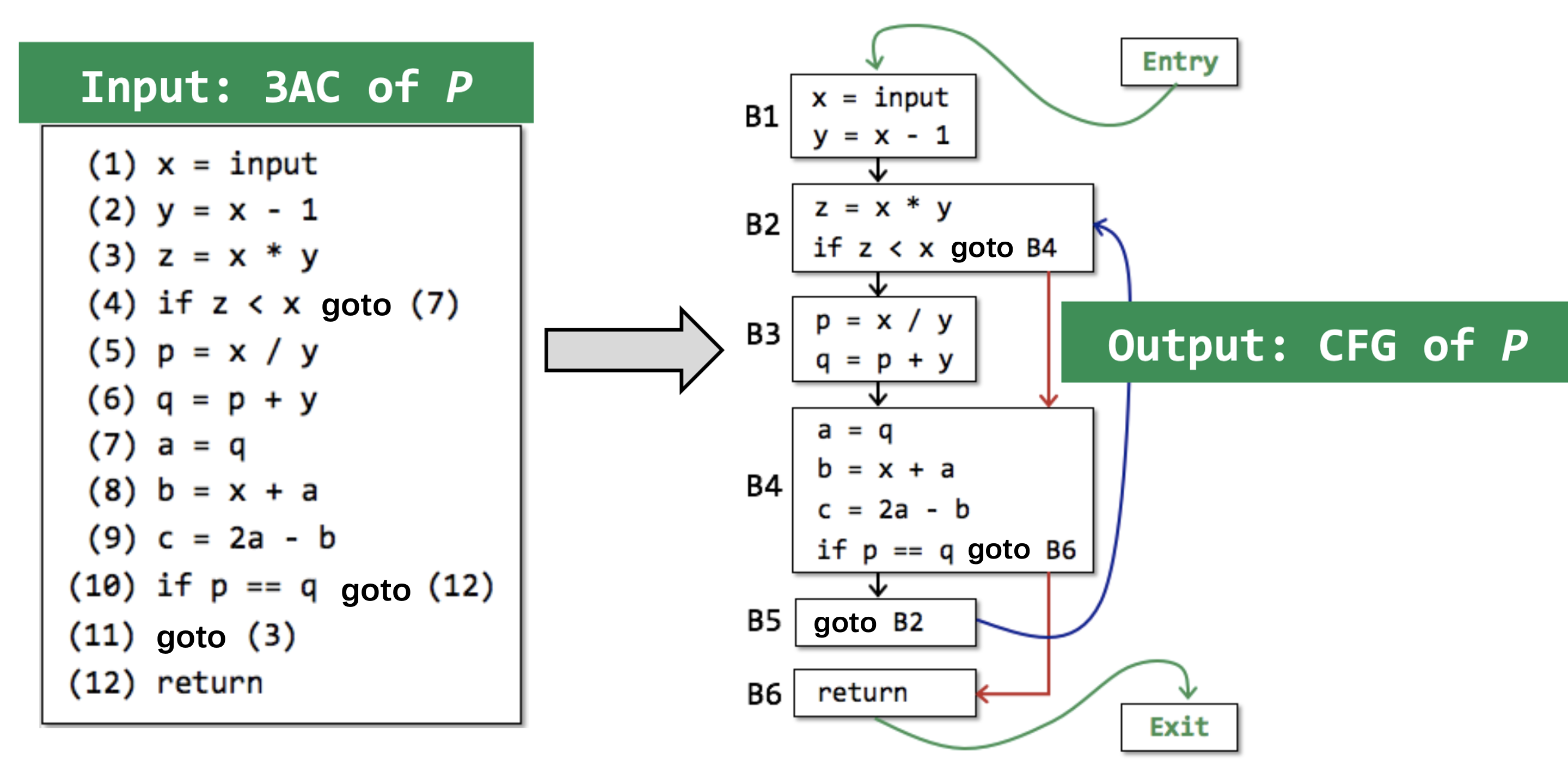# # 2 程序的中间表示

## # 2.1 编译器和静态分析器

### # 2.1.1 编程语言的各个层次• 扫描器（Scanner） 扫描源代码，进行词法分析（Lexical Analysis）,词法分析会用到正则表达式（Regular Expression），词法分析后的结果为一个标记（Token）串。

• 解析器（Parser） 遍历标记串，进行语法分析（Syntax Analysis），这里的语法分析分析的是上下文无关的语法（Context Free Grammer），解析器的内部应该是实现了一个有限状态机，用于识别和分析每个语法块格式的正确性，语法分析的结果为一棵抽象语法树（Abstract Syntax Tree, AST）。

• 类型检查器（Type Checker） 会遍历抽象语法树，进行语义分析（Semantic Analysis），不过编译器的语意分析是简单的，主要是分析属性语法（Attribute Grammer），比如说变量类型，并适当调整一下语法树。语义分析的结果我们称之为装饰过的抽象语法树（Decorated AST）。

• 翻译器（Translator） 会将抽象语法树翻译成中间表示（Intermediate Representation, IR），IR 的出现解耦了编译器的机器相关（Machine Dependent）部分和机器无关（Machine Independent）部分，上述几个层次在不同架构的机器上面是可以几乎不加改动地复用的。

• 机器码生成器（Code Generator） 会将 IR 转化成物理 CPU 能够直接执行的比特序列，这个过程是机器相关的。

### # 2.1.2 抽象语法树和中间表示的对比

do i = i + 1; while (a[i] < v);

1AST IR

### # 2.1.3 3地址码

• 名字（Name），包括

• 变量（Variable）

• 标签（Label）

• 用于指示程序位置，方便跳转指令的书写
• 字面常量（Literal Constant）

• 编译器生成的临时量（Compiler-Generated Temporary）

x = y bop z
x = uop y
x = y
goto L
if x goto L
if x rop y goto L

1
2
3
4
5
6

• x, y, z是变量的地址；

• bop 是双目操作符（Binary Operator），可以是算数运算符，也可以是逻辑运算符；

• uop 是单目操作符（Unary Operator），可能是取负、按位取反或者类型转换；

• L 是标签（Label），是标记程序位置的助记符，本质上还是地址；

• rop 是关系运算符（Relational Operator），运算结果一般为布尔值。

• goto 是无条件跳转， if... goto 是条件跳转。

### # 2.1.4 静态单赋值表示$x_3 = \phi(x_1, x_2) = \begin{cases} x_1, & \text{if true}\\ x_2, & \text{otherwise} \end{cases}$• 优点：

• 控制流的信息间接地被包含在了独特的变量名之中

• 当我们做一些对控制流敏感的分析的时候，这些信息可能会有帮助
• 定义和使用的关系明确

• 缺点：

• 可能会引入过多的变量名和$\phi$函数

• 被翻译成机器码的时候效率低，因为有太多对于程序执行来说不必要的赋值

## # 2.2 控制流分析### # 2.2.1 基块

CFG是我们进行静态分析的基础，控制流图中的结点可以是一个指令，也可以是一个基块（Basic Block）

• $IN_i = \{a_j | next(a_j) = a_i\}$ ，其中 $next(a_j)$ 表示控制流中 $a_j$ 的下一条指令；
• $OUT_i = \{a_j | prev(a_j) = a_i\}$ ，其中 $prev(a_j)$ 表示 $a_j$ 的上一条指令。

$\forall i \in [p + 1, q], IN_i = \{a_{i - 1}\} \wedge \forall i\in [p, q - 1], OUT_i = \{a_{i + 1}\}$

• 程序的控制流只能从首指令进入

• 程序的控制流只能从尾指令流出

$L = \{a_1\} \cup \{a_j | \exists a_i, type(a_i) = jump \wedge target(a_i) = a_j\} \cup \{a_{i + 1} | type(a_i) = jump\}$

• 整个程序的首指令；

• 跳转指令（包括条件跳转和无条件跳转）的目标指令；

• 跳转指令（包括条件跳转和无条件跳转）紧接着的下一条指令。

$a_p \in L \wedge a_{q + 1} \in L \wedge \forall a_i(i \in [p + 1, q]), a_i \notin L \Leftrightarrow \{a_p, a_{p + 1}, ..., a_{q - 1}, a_q\}\ is\ basic\ block$

### # 2.2.2 控制流图

$E_0 = \{(b_i, b_j) | (type(last(b_i)) = jump \wedge target(last(b_i)) = first(b_j)) \vee$
$(next(last(b_i)) = first(b_j)\wedge type(last(b_i))\ne unconditional\ jump) \}$

• 对于图 $CFG_0$ 中所有入度为0的点（一般只有一个），考虑虚拟结点 入口（Entry） ，所有的 入度（In Degree） 为0的点都是Entry的后继；

• 对于图 $CFG_0$ 中所有出度为0的点（可能不止一个），考虑虚拟结点 出口（Exit） ，所有的 出度（Out Degree） 为0的点都是Exit的前驱。

$E_1 = \{(Entry, b) | b \in B \wedge inDegree(b) = 0\},E_2 = \{(b, Exit) | b \in B \wedge outDegree(b) = 0\}$ 则我们可以定义 控制流图（Control Flow Graph）$CFG = (V, E)$ ，其中

$V = B \cup \{Entry, Exit\}, E = E_0 \cup E_1\cup E_2$

• 天然的顺序执行

• 这是计算系统天然存在的一种控制流
• 跳转指令

• 这是人为设计添加的一种控制流

## # 2.3 自检问题

1. 编译器（Compiler）和静态分析器（Static Analyzer）的关系是什么？